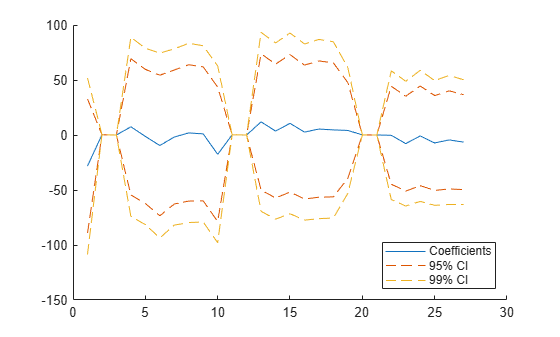# coefCI

Confidence intervals for coefficient estimates of multinomial regression model

Since R2023a

## Syntax

``ci = coefCI(mdl)``
``ci = coefCI(mdl,alpha)``

## Description

example

````ci = coefCI(mdl)` returns 95% confidence intervals for the coefficients in `mdl`. ```

example

````ci = coefCI(mdl,alpha)` returns the confidence intervals using the confidence level ```100(1 – alpha)```%. ```

## Examples

collapse all

Load the `carbig` data set.

`load carbig`

The variables `Horsepower`, `Weight`, and `Origin` contain data for car horsepower, weight, and country of origin, respectively. The variable `MPG` contains car mileage data.

Create a table in which the `Origin` and `MPG` variables are categorical.

```Origin = categorical(cellstr(Origin)); MPG = discretize(MPG,[9 19 29 39 48],"categorical"); tbl = table(Horsepower,Weight,Origin,MPG);```

Fit a multinomial regression model. Specify `Horsepower`, `Weight`, and `Origin` as predictor variables, and specify `MPG` as the response variable.

```modelspec = "MPG ~ 1 + Horsepower + Weight + Origin"; mdl = fitmnr(tbl,modelspec);```

Find the 95% confidence intervals for the coefficients. Display the coefficient names and confidence intervals in a table by using the `array2table` function.

```ci = coefCI(mdl); ciTable = array2table(ci, ... RowNames = mdl.Coefficients.Properties.RowNames, ... VariableNames = ["LowerLimit","UpperLimit"])```
```ciTable=27×2 table LowerLimit UpperLimit ___________ __________ (Intercept_[9, 19)) -89.395 32.927 Horsepower_[9, 19) 0.14928 0.27499 Weight_[9, 19) 0.0022537 0.0069061 Origin_France_[9, 19) -54.498 69.362 Origin_Germany_[9, 19) -62.237 59.666 Origin_Italy_[9, 19) -73.457 54.35 Origin_Japan_[9, 19) -62.743 59.097 Origin_Sweden_[9, 19) -60.076 63.853 Origin_USA_[9, 19) -59.875 61.926 (Intercept_[19, 29)) -78.671 43.544 Horsepower_[19, 29) 0.12131 0.24115 Weight_[19, 29) -0.00073846 0.0033281 Origin_France_[19, 29) -49.929 73.841 Origin_Germany_[19, 29) -57.315 64.476 Origin_Italy_[19, 29) -51.881 73.071 Origin_Japan_[19, 29) -58.22 63.559 ⋮ ```

Each row contains the lower and upper limits for the 95% confidence intervals.

Load the `carbig` data set.

`load carbig`

The variables `Horsepower`, `Weight`, and `Origin` contain data for car horsepower, weight, and country of origin. The variable `MPG` contains car mileage data.

Create a table in which the `Origin` and `MPG` variables are categorical.

```Origin = categorical(cellstr(Origin)); MPG = discretize(MPG,[9 19 29 39 48],"categorical"); tbl = table(Horsepower,Weight,Origin,MPG);```

Fit a multinomial regression model. Specify `Horsepower`, `Weight`, and `Origin` as predictor variables, and specify `MPG` as the response variable.

```modelspec = "MPG ~ 1 + Horsepower + Weight + Origin"; mdl = fitmnr(tbl,modelspec);```

Find the 95% and 99% confidence intervals for the coefficients. Display the coefficient names and confidence intervals in a table by using the `array2table` function.

```ci95 = coefCI(mdl); ci99 = coefCI(mdl,0.01); confIntervals = array2table([ci95 ci99], ... RowNames=mdl.Coefficients.Properties.RowNames, ... VariableNames=["95LowerLimit","95UpperLimit", ... "99LowerLimit","99UpperLimit"])```
```confIntervals=27×4 table 95LowerLimit 95UpperLimit 99LowerLimit 99UpperLimit ____________ ____________ ____________ ____________ (Intercept_[9, 19)) -89.395 32.927 -108.66 52.194 Horsepower_[9, 19) 0.14928 0.27499 0.12948 0.29478 Weight_[9, 19) 0.0022537 0.0069061 0.0015209 0.0076389 Origin_France_[9, 19) -54.498 69.362 -74.007 88.871 Origin_Germany_[9, 19) -62.237 59.666 -81.438 78.868 Origin_Italy_[9, 19) -73.457 54.35 -93.588 74.481 Origin_Japan_[9, 19) -62.743 59.097 -81.935 78.288 Origin_Sweden_[9, 19) -60.076 63.853 -79.596 83.373 Origin_USA_[9, 19) -59.875 61.926 -79.06 81.111 (Intercept_[19, 29)) -78.671 43.544 -97.921 62.794 Horsepower_[19, 29) 0.12131 0.24115 0.10243 0.26003 Weight_[19, 29) -0.00073846 0.0033281 -0.001379 0.0039687 Origin_France_[19, 29) -49.929 73.841 -69.424 93.336 Origin_Germany_[19, 29) -57.315 64.476 -76.498 83.659 Origin_Italy_[19, 29) -51.881 73.071 -71.563 92.752 Origin_Japan_[19, 29) -58.22 63.559 -77.401 82.74 ⋮ ```

Each row contains the lower and upper limits for the 95% and 99% confidence intervals.

Visualize the confidence intervals by plotting their limits with the coefficient values.

```ci95 = coefCI(mdl); ci99 = coefCI(mdl,0.01); colors = lines(3); hold on p = plot(mdl.Coefficients.Value,Color=colors(1,:)); plot(ci95(:,1),Color=colors(2,:),LineStyle="--") plot(ci95(:,2),Color=colors(2,:),LineStyle="--") plot(ci99(:,1),Color=colors(3,:),LineStyle="--") plot(ci99(:,2),Color=colors(3,:),LineStyle="--") hold off legend(["Coefficients","95% CI","","99% CI",""], ... Location="southeast")```The plot shows that the 99% confidence intervals for the coefficients are wider than the 95% confidence intervals.

## Input Arguments

collapse all

Multinomial regression model object, specified as a `MultinomialRegression` model object created with the `fitmnr` function.

Significance level for the confidence interval, specified as a numeric value in the range [0,1]. The confidence level of `ci` is equal to ```100(1 – alpha)```%. `alpha` is the probability that the confidence interval does not contain the true value.

Example: `0.01`

Data Types: `single` | `double`

## Output Arguments

collapse all

Confidence intervals, returned as a p-by-2 numeric matrix, where p is the number of coefficients. The jth row of `ci` is the confidence interval for the jth coefficient of `mdl`. The name of coefficient j is stored in the `CoefficientNames` property of `mdl`.

collapse all

### Confidence Intervals

The coefficient confidence intervals provide a measure of precision for regression coefficient estimates.

A 100(1 – α)% confidence interval gives the range for the corresponding regression coefficient with 100(1 – α)% confidence, meaning that 100(1 – α)% of the intervals resulting from repeated experimentation will contain the true value of the coefficient.

The software finds confidence intervals using the Wald method. The 100(1 – α)% confidence intervals for regression coefficients are

`${b}_{i}±{t}_{\left(1-\alpha /2,n-p\right)}SE\left({b}_{i}\right),$`

where bi is the coefficient estimate, SE(bi) is the standard error of the coefficient estimate, and t(1–α/2,np) is the 100(1 – α/2) percentile of the t-distribution with n – p degrees of freedom. n is the number of observations and p is the number of regression coefficients.

## Version History

Introduced in R2023a